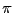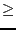Next: Examples Up: Solving trigonometric equation Previous: Mathematical background   Contents

Implementation

The purpose of this procedure is to determine the roots of a trigonometric equation within a given angle interval.

int Solve_Trigo_Interval(int n,VECTOR &A,INTEGER_VECTOR &SSin,
INTEGER_VECTOR &CCos,double epsilon,double epsilonf,
int M,int Stop,INTERVAL_VECTOR &Solution,int Nb,REAL Inf,REAL Sup);
with:
• M: the maximum number of boxes which may be stored. See the note 2.3.4.5
• Stop: the possible values are 0,1,2
• 0: the algorithm will look for every solution in the angle interval
• 1: the algorithm will stop as soon as 1 solution has been found
• 2: the algorithm will stop as soon as Nb solutions have been found
• epsilon: the maximal width of the box, see the note 2.3.4.6
• epsilonf: the maximal width of the equation intervals, see the note 2.3.4.6
• Solution: an interval vector of size at least Nb which will contained the solution intervals.
• Nb: the maximal number of solution which will be returned by the algorithm
• Inf, Sup: the bound of the angle interval in which we are looking for solutions.
Note that the returned Solution will always be a range [a,b] included in [0,2] and in [Inf,Sup], this interval angle being reduced to an angle interval in the range [0,2]. The procedure will return:
•0: the number of roots
• -1: the bound Inf or Sup is incorrect (positive or negative infinity)
If you are looking for all the roots of the trigonometric equation you may either specify [Inf,Sup] as [0,2] or use the procedure:

int Solve_Trigo_Interval(int n,VECTOR &A,INTEGER_VECTOR &SSin,
INTEGER_VECTOR &CCos,double epsilon,double epsilonf,
int M,int Stop,INTERVAL_VECTOR &Solution,int Nb);
This procedure first analyze the trigonometric equation to find bounds on the roots using the algorithm described in section 4.3, then use the previous procedure to determine the roots within the bound. In some case this procedure may be faster than the general purpose algorithm.Next: Examples Up: Solving trigonometric equation Previous: Mathematical background   Contents
Jean-Pierre Merlet 2012-12-20